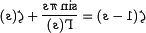# Riemann Hypothesis

(Redirected from Riemann hypothesis)
Award of Confusion!

Apparently this article is damned funny. It is not an inside joke or vanity page, but you pretty much need a PhD to get it. Or so we've been told, most of us seem to prefer humour involving masturbation and/or Jesus. The author may have been masturbating over a picture of Jesus. You can send him to hell by giving him an atomic fisting.

For those without comedic tastes, the so-called experts at Wikipedia have an article about Riemann Hypothesis.

The Riemann Hypothesis was a key mathematical conjecture in the field of theoretical gangsta rap which remained unsolved for centuries. It concerns the behavior of the quality function in any given song, predicting where and when it will hit rock bottom (or zero, as mathematicians call it to appear smarter than they actually are). Of course, as it regards rap music, the quality is purely imaginary.

The importance of this conjecture for number theory and cryptography led the Clay Institute to offer a million-dollar prize for its solution. It was finally proven in 2004 by none other than Chuck Norris, famous logician, mathematician and finger-painter. His remarkably simple and direct proof is presented further on in this article.

## Profound Meaning

For many years, mathematicians have puzzled over the hypothesis, first presented by Optimus "Riemann" Rhyme in the early 1980s.

In one of its equivalent formulations, it states that:

• No matter which word you end a line of a song with, there will always exist a word that rhymes with it for use in future lines.

This was obviously of great importance back in the days of old skool rap and is almost certain to be partially responsible for Optimus' meteoric rise to fame. Songwriters are still looking for the word that rhymes with rainbow.

## Skeptics

There are those who choose to disbelieve the hypothesis. Attempts to disprove it began in 1981 when The Sugarhill Gang released a controversial track with the ending line "Like an orange". This clear challenge to the theory, from such prominent mathematicians, took the world of mathematics by storm.

It wasn't until ten years later in 1991 that the creativity of De La Soul, behind the theory that three is a magic number, released a response album entitled “Orange, so sorange, why don't you get off my morange?”, thereby showing that the Sugarhill Gang had failed to provide a counter-example. The full proof, however, had to await another decade, and the genius of Chuck Norris.

## The First Elementary Proof

Here we describe, briefly, Norris's proof of the conjecture. We assume the reader has basic knowledge of analytic number theory and pottery.

Theorem: In any single piece of rap music, the imaginary quality of the music is a function of how stoned the writer is, $\zeta (s)$. The quality hits rock bottom (zero) when the writer is exactly HALF stoned: too far out to produce coherent stuff, and too sober to access his subconscious genius.

Proof: In the begining, there is an L-function, which looks like this:

$\zeta (s)=\sum _{n}^{\infty }{\frac {L}{n^{s}}}$When L=1, then every zero has s=1/2 which can be shown using following easy proof:

$\zeta (s+1)-\zeta (s)=\sum _{n}^{\infty }{\frac {1}{(n+1)^{s}}}-\sum _{n}^{\infty }{\frac {1}{n^{s}}}={\frac {1}{n^{s}}}$Substituting n=1/2 into formula and solving for s, one gets

$\zeta (s+1)-\zeta (s)=2^{s}$According to the reflection theorem, one has

$\zeta (1-s)={\frac {\sin \pi s}{\Gamma (s)}}+\zeta (s)$and alsowhich means

$\zeta (s+1)={\sqrt {2}}+\zeta (s)$Substituting into the above, means that

${\sqrt {2}}=2^{s}$is what we wanted to show, that s=1/2. This is the easiest proof of the Riemann Hypothesis, which can be proven in other ways. In 1900 David Hilbert included the Riemann Hypothesis in his list of the 23 simplest and least mathematically significant problems.